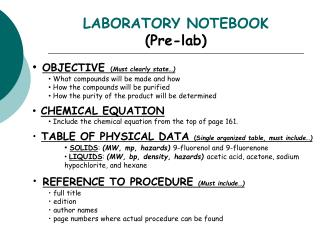DownloadDownload PresentationLABORATORY NOTEBOOK (Pre-lab)

# LABORATORY NOTEBOOK (Pre-lab)

Download Presentation## LABORATORY NOTEBOOK (Pre-lab)

- - - - - - - - - - - - - - - - - - - - - - - - - - - E N D - - - - - - - - - - - - - - - - - - - - - - - - - - -
##### Presentation Transcript

1. LABORATORY NOTEBOOK(Pre-lab) • OBJECTIVE(Must clearly state…) • What compounds will be made and how • How the compounds will be purified • How the purity of the product will be determined • CHEMICAL EQUATION • Include the chemical equation from the top of page 161. • TABLE OF PHYSICAL DATA (Single organized table, must include…) • SOLIDS: (MW, mp, hazards) 9-fluorenol and 9-fluorenone • LIQUIDS: (MW, bp, density, hazards) acetic acid, acetone, sodium hypochlorite, and hexane • REFERENCE TO PROCEDURE (Must include…) • full title • edition • author names • page numbers where actual procedure can be found

2. LABORATORY NOTEBOOK(In-lab) • DATA/CALCULATIONS • Initial weight of 9-fluorenol used • TLC diagram (s) with all cm measurements • Initial weight of 150 mL beaker • Final weight of 150 mL beaker + product • Final product weight • Physical state and color of product • Theoretical yield calculation • Percent yield calculation • Atom economy calculation • Cost per synthesis and cost per gram calculations • Give at least one TLC Rf value calculation • EXPERIMENTAL PROCEDURE • In paragraph form, describe the procedure that you actually followed during the lab. • Paragraph must be written in PAST TENSE, PASSIVE VOICE. • Include any volumes or weights of chemicals used during the experiment. • Include any observations or errors that occur (if any).